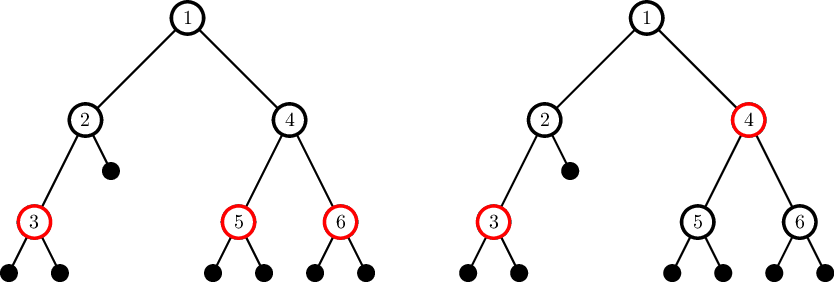# Second qualification round completed

## Tasks

Show one task per page / all tasks on one page

"D" Red-Black Tree
Time limit 3 seconds 256 megabytes

Did you now that most standard libraries use red-black tree to implement "set" data structure? In this problem you have to find the number of ways to color the vertices of the given binary tree so that it became red-black. Print the answer modulo 109 + 7.

Consider a binary tree. If the vertex has less than two children, add fake vertices to the potential places of the missing children. The tree is called a red-black tree if the following constraints are satisfied:

1. Each vertex is colored one of the two colors: red or black.
2. The root of the tree and added fake vertices are colored black.
3. The parent of a red vertex is black.
4. All paths the root to fake leafs contain the same number of black vertices.

Note that the parent of a black vertex can be black itself.

Two ways to color the tree are different if there is a vertex that has different colors.

The picture shows two ways to color the tree from the second test case.Input format

The first line contains one integer n — the number of vertices in a tree (1 ≤ n ≤ 500 000).

The following n lines describe a tree. The i-th of these lines contains two integers li and ri — the indices of the left and the right child of the i-th vertex, or 0 if the corresponding child is missing (li = 0 or i < li ≤ n; ri = 0 or i < ri ≤ n). The root of the tree has number 1. Input describes the correct tree.

Output format

Output one integer — the number of ways to color the given tree so that it was a correct red-black tree. The answer must be printed modulo 109 + 7.

Examples
Input data
```3
2 3
0 0
0 0
```
Output data
```2
```
Input data
```6
2 4
3 0
0 0
5 6
0 0
0 0
```
Output data
```2
```

### Send solution

Maximal size is 256kb

### Log in

The instruction for password recovery
has been sent to your email